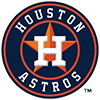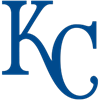2022 AL Cy Young Odds
+500
5 to 1Justin Verlander
11.5% implied probability

+650
6.5 to 1Kevin Gausman
9.2% implied probability

+750
7.5 to 1Gerrit Cole
8.1% implied probability

+800
8 to 1Dylan Cease
7.7% implied probability

+900
9 to 1Alek Manoah
6.9% implied probability

+1000
10 to 1Shohei Ohtani
6.3% implied probability

+1000
10 to 1Shane McClanahan
6.3% implied probability

+1400
14 to 1Nestor Cortes
4.6% implied probability

+1500
15 to 1Shane Bieber
4.3% implied probability

+2000
20 to 1Logan Gilbert
3.3% implied probability

+2000
20 to 1Lucas Giolito
3.3% implied probability

+3500
35 to 1Noah Syndergaard
1.9% implied probability

+4000
40 to 1Robbie Ray
1.7% implied probability

+4000
40 to 1Michael Kopech
1.7% implied probability

+5000
50 to 1Luis Severino
1.4% implied probability

+5000
50 to 1Joe Ryan
1.4% implied probability

+5000
50 to 1Patrick Sandoval
1.4% implied probability

+5000
50 to 1Framber Valdez
1.4% implied probability

+5000
50 to 1Frankie Montas
1.4% implied probability

+5000
50 to 1Tarik Skubal
1.4% implied probability

+8000
80 to 1Jose Berrios
0.9% implied probability

+8000
80 to 1Paul Blackburn
0.9% implied probability

No changes have been recorded yet.
+8000
80 to 1Triston McKenzie
0.9% implied probability

+10000
100 to 1Jose Urquidy
0.7% implied probability

+10000
100 to 1Casey Mize
0.7% implied probability

+10000
100 to 1Luis Garcia
0.7% implied probability

+10000
100 to 1Lance Lynn
0.7% implied probability

+10000
100 to 1Brad Keller
0.7% implied probability

+10000
100 to 1Hyun Jin Ryu
0.7% implied probability

+10000
100 to 1Jordan Montgomery
0.7% implied probability

+10000
100 to 1Lance McCullers
0.7% implied probability

+12500
125 to 1Dylan Bundy
0.5% implied probability

+12500
125 to 1Corey Kluber
0.5% implied probability

+15000
150 to 1Sonny Gray
0.5% implied probability

+15000
150 to 1Aroldis Chapman
0.5% implied probability

No changes have been recorded yet.
+15000
150 to 1Nathan Eovaldi
0.5% implied probability

+15000
150 to 1Chris Sale
0.5% implied probability

+15000
150 to 1Eduardo Rodriguez
0.5% implied probability

+15000
150 to 1Jon Gray
0.5% implied probability

+15000
150 to 1Aaron Civale
0.5% implied probability

+15000
150 to 1Shane Baz
0.5% implied probability

+15000
150 to 1Michael King
0.5% implied probability

No changes have been recorded yet.
+20000
200 to 1Chris Flexen
0.3% implied probability

+20000
200 to 1Jake Odorizzi
0.3% implied probability

+20000
200 to 1Marco Gonzales
0.3% implied probability

+20000
200 to 1Jameson Taillon
0.3% implied probability

+20000
200 to 1Yusei Kikuchi
0.3% implied probability

+20000
200 to 1Zach Plesac
0.3% implied probability